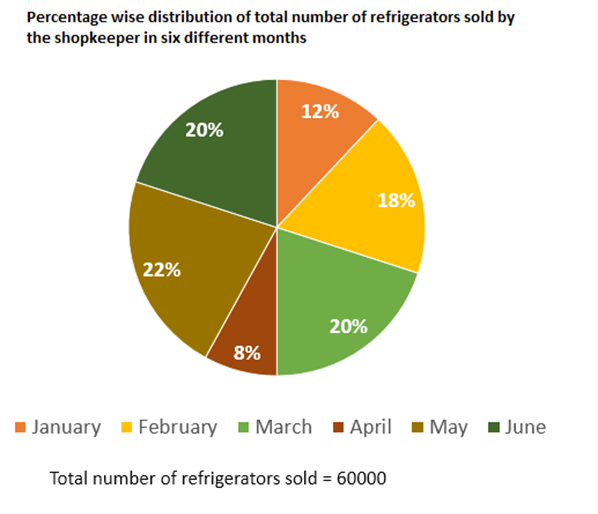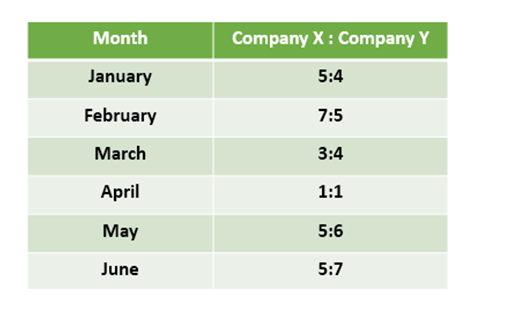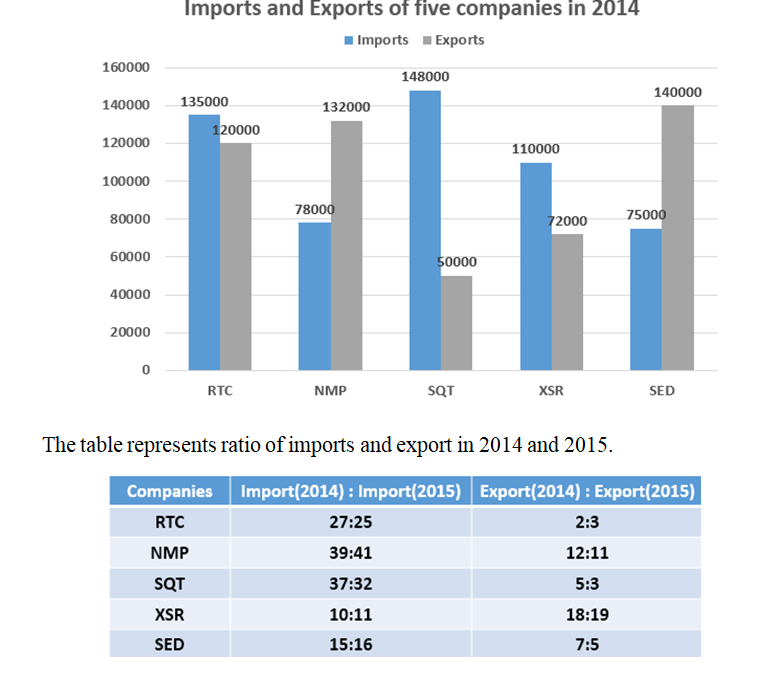# Quantitative Aptitude Questions (Data Interpretation) for SBI Clerk / IDBI Executive 2018 Day-74

Dear Readers, SBI is conducting Online preliminary Examination for the recruitment of Clerical Cadre. preliminary Examination of SBI Clerk was scheduled from June 2018. To enrich your preparation here we have providing new series of Data Interpretation – Quantitative Aptitude Questions. Candidates those who are appearing in SBI Clerk Prelims and IDBI Executive Exams can practice these Quantitative Aptitude average questions daily and make your preparation effective.

[WpProQuiz 2066]

Click “Start Quiz” to attend these Questions and view Solutions

Directions (Q. 1 – 5): Study the following information carefully and answer the questions given below:1. Number of refrigerators of company X sold by the shopkeeper in January and February together is approximately what percent of total number refrigerators sold by the shopkeeper in March?

a) 78 %

b) 86 %

c) 74 %

d) 92 %

e) 69 %

1. Find the respective ratio of total number of refrigerators of company Y sold by the shopkeeper in February to that of in April.

a) 15:8

b) 17:9

c) 8:15

d) 9:17

e) None of these

1. Find the difference between number of refrigerators of company X sold by the shopkeeper in June and number of refrigerators of company Y sold by the shopkeeper in May.

a) 2600

b) 2400

c) 2200

d) 2300

e) None of these

1. Find the total number of refrigerators of company X sold by the shopkeeper in January, February, May and June.

a) 13200

b) 21300

c) 23100

d) 12300

e) None of these

1. Total number of refrigerators sold by the shopkeeper in March is what percent more than the number of refrigerators of company Y sold by the shopkeeper in January?

a) 255 %

b) 265 %

c) 235 %

d) 275 %

e) None of these

Directions (Q. 6 – 10) Study the following information carefully and answer the questions given below:

The following bar graph represents the exports (in Rs.) and imports (in Rs.) of five companies in 20141. Find the respective ratio between Import of SQT in 2015 and export of XSR in 2015.

a) 37:18

b) 32:19

c) 18:37

d) 19:32

e) None of these

1. Imports of all the companies together in 2014 is what percent of the exports of all the companies together in 2014?

a) 128.44 %

b) 94.88 %

c) 112.66 %

d) 106.22 %

e) 88.44 %

1. Find the sum of the imports of the companies RTC and NMP in 2015 and exports of the companies SQT and XSR in 2015.

a) Rs. 423000

b) Rs. 313000

c) Rs. 396000

d) Rs. 203000

e) Rs. 412000

1. Find the difference between the sum of Import and Export of company SED in 2014 and that in 2015.

a) Rs.20000

b) Rs.35000

c) Rs.18000

d) Rs.21000

e) None of these

1. Import of company SQT in 2015 is approximately what percent more/less than the export of the same company in same year?

a) 295 %

b) 396 %

c) 327 %

d) 418 %

e) 243 %

Directions (Q. 1 – 5):

Explanation:

Number of refrigerators of company X sold by the shopkeeper in January and February together

= (5/9) x (12/100) x 60000 + (7/12) x (18/100) x 60000

= 4000 + 6300

= 10300

Total number of refrigerators sold by the shopkeeper in March = 20/100 x 60000 = 12000

Required percentage = (10300/12000) x 100

= 85.83% = 86 %

Explanation:

Total number of refrigerators of company Y sold by the shopkeeper in February

= (5/12) x (18/100) x 10800

= 4500

Total number of refrigerators of company Y sold by the shopkeeper in April

= (½) x (8/100) x 60000

= 2400

Required ratio = 4500 : 2400 = 15:8

Explanation:

Number of refrigerators of company X sold by the shopkeeper in June

= (5/12) x (20/100) x 60000

= 5000

Number of refrigerators of company Y sold by the shopkeeper in May

= (6/11) x (22/100) x 60000

= 7200

Required difference = 7200 – 5000 = 2200

Explanation:

Total number of refrigerators of company X sold by the shopkeeper in January, February, May and June

= (5/9) x (12/100) x 60000 + (7/12) x (18/100) x 60000 + (5/11) x (22/100) x 60000 + (5/12) x (20/100) x 60000

= 4000 + 6300 + 6000 + 5000

= 21300

Explanation:

Total number of refrigerators sold by the shopkeeper in March

= (20/100) x 60000 = 12000

Number of refrigerators of company Y sold by the shopkeeper in January

= (4/9) x (12/100) x 60000

= 3200

Required percentage = [(12000 – 3200)/3200] x 100 = 275 %

Directions (Q. 6 – 10)

Explanation:

Import of SQT in 2015 = (32/37) x 148000 = Rs.128000

Export of XSR in 2015 = (19/18) x 72000 = Rs.76000

Required ratio = 128000 : 76000 = 32:19

Explanation:

Imports of all the companies together in 2014

= 135000 + 78000 + 148000 + 110000 + 75000

= Rs.546000

Exports of all the companies together in 2014

= 120000 + 132000 + 50000 + 72000 + 140000

= Rs.514000

Required percentage = (546000/514000) x 100 = 106.22 %

Explanation:

Import of RTC in 2015 = (25/27) x 135000 = Rs.125000

Import of NMP in 2015 = (41/39) x 78000 = Rs.82000

Export of SQT in 2015 = (3/5) x 50000 = Rs.30000

Export of XSR in 2015 = (19/18) x 72000 = Rs.76000

Required sum = 125000 + 82000 + 30000 + 76000 = Rs. 313000

Explanation:

Sum of Import and Export of company SED in 2014

= 75000 + 140000 = Rs. 215000

Sum of Import and Export of company SED in 2015

= 16/15 x 75000 + 5/7 x 140000

= 80000 + 100000

= Rs. 180000

Required difference = 215000 – 180000 = Rs. 35000

Explanation:

Import of SQT in 2015 = (32/37) x 148000 = Rs.128000

Export of SQT in 2015 = (3/5) x 50000 = Rs.30000

Required percentage = [(128000 – 30000)/30000] x 100

= (98000/30000) x 100

= 326.67%

= 327 %

Daily Practice Test Schedule | Good Luck

 Topic Daily Publishing Time Daily News Papers & Editorials 8.00 AM Current Affairs Quiz 9.00 AM Logical Reasoning 10.00 AM Quantitative Aptitude “20-20” 11.00 AM Vocabulary (Based on The Hindu) 12.00 PM Static GK Quiz 1.00 PM English Language “20-20” 2.00 PM Banking Awareness Quiz 3.00 PM Reasoning Puzzles & Seating 4.00 PM Daily Current Affairs Updates 5.00 PM Data Interpretation / Application Sums (Topic Wise) 6.00 PM Reasoning Ability “20-20” 7.00 PM English Language (New Pattern Questions) 8.00 PM General / Financial Awareness Quiz 9.00 PM